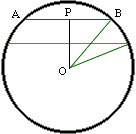Date: Sun, 27 Sep 1998 11:42:59 -0600 (CST)

Name: Jennifer

 In the diagram OBP is a right angled triangle with |OB| = 21/2 cm, and |BP| = 10/2 cm so |OP|^2 = |OB|^2 - |BP|^2 = 441/4 - 100/4 = 341/4 cm^2. Thus |OP| = Sqrt(341/4) cm. Using the same technique you can find the distance from the center of the circle to the chord BC and then a subtraction gives the distance you want. Cheers, Harley